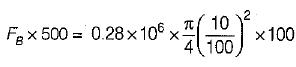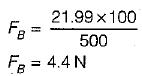Courses

# Test: Buoyancy & Floatation - 2

## 10 Questions MCQ Test Topicwise Question Bank for Mechanical Engineering | Test: Buoyancy & Floatation - 2

Description
This mock test of Test: Buoyancy & Floatation - 2 for Mechanical Engineering helps you for every Mechanical Engineering entrance exam. This contains 10 Multiple Choice Questions for Mechanical Engineering Test: Buoyancy & Floatation - 2 (mcq) to study with solutions a complete question bank. The solved questions answers in this Test: Buoyancy & Floatation - 2 quiz give you a good mix of easy questions and tough questions. Mechanical Engineering students definitely take this Test: Buoyancy & Floatation - 2 exercise for a better result in the exam. You can find other Test: Buoyancy & Floatation - 2 extra questions, long questions & short questions for Mechanical Engineering on EduRev as well by searching above.
QUESTION: 1

### When a body, floating in a liquid is given a small angular displacement, it starts oscillating about a point known as

Solution: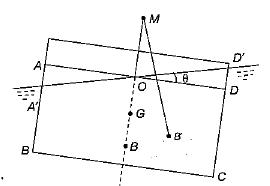Where M is metacentre and θ is small angle of hill through which a body is tilted.
Hence metacenter is a point about which a body oscillate when tilted or given a small angular displacement.

QUESTION: 2

### The time of oscillation of a floating body is given by Where K = Radius of Gyration of the floating body about its centre of gravity h = Metacentric height of the floating body

Solution:

Time of oscillation of a floating body is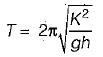h = metracentric height

QUESTION: 3

### The time of oscillation of a floating body with increase in metacentric height will be

Solution:

Time of oscillation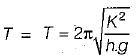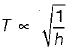as h increases, time of oscillation decreases

QUESTION: 4

A floating body is in stable equilibrium when

Solution:

For stable equilibrium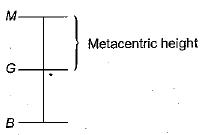QUESTION: 5

A submerged body will be in stable equillibrium. if

Solution:

For stable equilibrium of submerged body: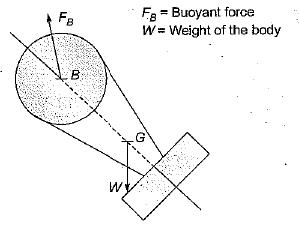Hence for stable equilibrium of submerged body centre of buoyancy B should be above G.

QUESTION: 6

Which one of the following is the conditions for stable equilibrium for a floating body

Solution:

For stable equilibrium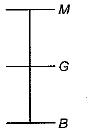For stable equilibrium metacenter is above the center of gravity.

QUESTION: 7

How is the metacentric height (GM) expressed
Where I = Moment of inertia of the plan of the floating body at the water surface
V = Volume of the body submerged in water
BG = Distance between the center of gravity (G) and the center of Buoyancy (B)

Solution: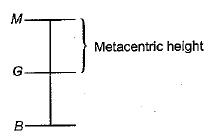Metacentric height,
GM = BM - BG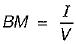Where I = Area moment of inertia of top view about longitudinal axis
V = Volume of the fluid displaced
Hence,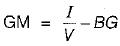QUESTION: 8

An odd shaped body weighing 7.5 kg and occupying 0.01 m3 volume wiil be completely submerged in a fluid having specific gravity of

Solution:
QUESTION: 9

A cylindrical body of cross-sectional area A height H and density ρs is immersed to a depth h in a liquid of density ρ and tied down to bottom with a string then the tension in the string is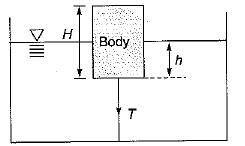Solution:

w + T =  FB = weight of fluid displaced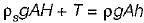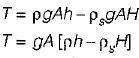QUESTION: 10

A float valve of the ‘ball-cock’ type required to close an opening of a supply pipe feeding a cistern as shown in the given figure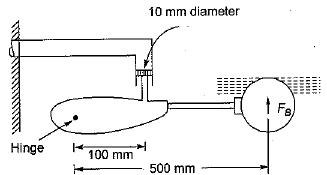The buoyant force FB required to be exerted by the float to keep the valve closed against a pressure of 0.28 N/mm2 is

Solution:

Taking moment about hinge We get